Author: Oscar Cronquist Article last updated on February 07, 2023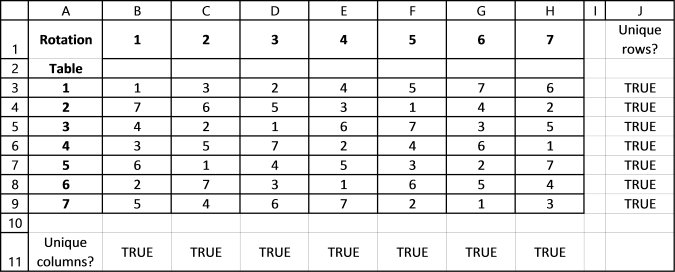Kristina asks:Hi Oscar,Your formula works great, however, I was wondering if there is capability to add another countif criteria so that it produces a random unique number different from the one above AS WELL AS the one to the left.I have 7 groups, 7 tables, and 7 rotations for which each group will move to a different table.

With your formula, I am able to successfully assign each group a random order of the 7 tables to rotate too with no repeats (however I am unable to guarantee that for each rotation only one group is at each table), OR I am able to produce a schedule that has max one group per table per rotation, but then I am not able to have each group have no repeats for the tables they are rotating too.

Is there a way to tweak the formula to satisfy both requirements where each group has a random 1-7 table rotation, AND for each rotation there is only one group per table? Thanks!! Appreciate the help.

This is not as easy as it may seem. I first tried using the same technique as in the post but the formula returned errors which is really not surprising if you think of it. I will explain why later in this post but first you need to know how the original formula works.

The original formula uses the COUNTIF function to take previous values vertically into account and return a new unique random number between 1 and 7. This works well, unique random numbers between 1 to 7 are produced in column B.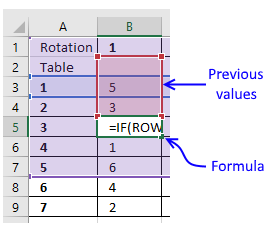Array formula in cell B5:

=IF(ROW(3:3)<8, LARGE((COUNTIF(\$B\$2:B4, ROW(\$1:\$7))=0)*ROW(\$1:\$7), RANDBETWEEN(1, SUM(--(COUNTIF(\$B\$2:B4, ROW(\$1:\$7))=0)))), "")

I tweaked the formula so it also considers previous values horizontally to make sure a table never has a duplicate group number, see picture below.Here is the entire table, the formulas return almost always a #num error somewhere in the table. Press F9 to recalculate values if you have the attached workbook open.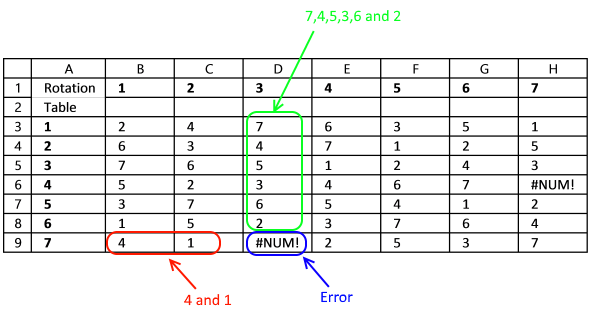So why is this happening? Look at cell D9, number 4 and 1 is to the left of cell D9. This means that only numbers 2,3,5,6 or 7 can be populated in cell D9 or there will be a duplicate on row 9.

In this case, the values above cell D9 are 7,4,5,3,6 and 2 so the only value left is 1 or there will be a duplicate in column D. Number 1 is not possible in cell D9 so the formula returns a #NUM error.

The formulas above cell D9 do not take this into consideration while producing a random value, they only look back at previous values. It is not possible for the formulas to look at cells below or to the right because excel calculates each cell in this order.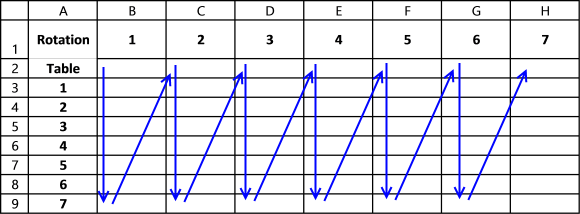It begins with the upper most left cell containing a formula, in this case B2 and then continues with the next cell below and so on, until there are no more formulas in that column. Excel then continues with the first formula in the next column, in this case cell C2.

## This problem needs a new approach

My second attempt to solve this problem is to move each value one cell for each rotation, the picture below shows how in the second rotation.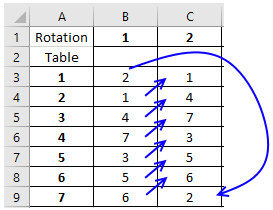The problem with this approach is that only the first rotation has random values, the remaining rotations are not random. However, this technique makes it absolutely certain that a table has no repeats. Perhaps it is random enough?

Formula in cell C3:

=INDEX(\$B\$3:\$B\$9, IF(MATCH(B3, \$B\$3:\$B\$9, 0)=7, 1, MATCH(B3, \$B\$3:\$B\$9, 0)+1))

To make it even more random I changed the formula so the first value in each column is random (row 3) but the sequence is the same as in rotation 1 (column B). As before, it takes previous values horizontally into account when calculating a new unique value.Array formula in cell B3:

=IF(ROW(1:1)<8, LARGE((COUNTIF(\$B\$2:B2, ROW(\$1:\$7))=0)*ROW(\$1:\$7), RANDBETWEEN(1, SUM(--(COUNTIF(\$B\$2:B2, ROW(\$1:\$7))=0)))), "")

Copy cell B3 and paste to cell range B4:B9.

### Explaining formula in cell B3

#### Step 1 - Create array containing a sequence from 1 to 7

The ROW function returns a number representing the row number of a cell reference.

ROW(\$1:\$7)

returns {1; 2; 3; 4; 5; 6; 7}.

#### Step 2 - Count numbers in column B based on sequence 1 to 7

This step checks that previous cells above is not repeated again.

Cell reference \$B\$2:B2 grows when cell B3 is copied and pasted to cells below.

The COUNTIF function counts cells that are equal to a condition or criteria.

COUNTIF(\$B\$2:B2, ROW(\$1:\$7))

becomes

COUNTIF(\$B\$2:B2, {1; 2; 3; 4; 5; 6; 7})

becomes

COUNTIF(0, {1; 2; 3; 4; 5; 6; 7})

and returns {0; 0; 0; 0; 0; 0; 0}.

#### Step 3 - Check if number in array is erqual to 0 (zero)

The equal sign compares the values in the array to 0 (zero), the result is either TRUE or FALSE.

COUNTIF(\$B\$2:B2, ROW(\$1:\$7))=0

becomes

{0; 0; 0; 0; 0; 0; 0}=0

and returns {TRUE; TRUE; TRUE; TRUE; TRUE; TRUE; TRUE}.

#### Step 4 - Convert boolean value to numerical value

The SUM function is not able to add boolean values, we need to convert the boolean values to their numerical equivalents. TRUE = 1 and FALSE = 0 (zero).

--(COUNTIF(\$B\$2:B2, ROW(\$1:\$7))=0)

becomes

--({TRUE; TRUE; TRUE; TRUE; TRUE; TRUE; TRUE})

and returns {1; 1; 1; 1; 1; 1; 1}.

#### Step 5 - Sum numerical values

The SUM function adds numerical values and ignores text and blanks, it then returns the total.

SUM(--(COUNTIF(\$B\$2:B2, ROW(\$1:\$7))=0))

becomes

SUM({1; 1; 1; 1; 1; 1; 1})

and returns 7.

#### Step 6 - Create a raandom number between 1 and the sum

The RANDBETWEEN function returns a number between a bottom number and a top number.

RANDBETWEEN(bottomtop)

RANDBETWEEN(1, SUM(--(COUNTIF(\$B\$2:B2, ROW(\$1:\$7))=0)))

becomes

RANDBETWEEN(1, 7)

and returns 7 (or any number between 1 and 7).

#### Step 7 - Extrakt the k-th largest number

The LARGE function returns the k-th largest number ina cell range or array.

LARGE(arrayk)

LARGE((COUNTIF(\$B\$2:B2, ROW(\$1:\$7))=0)*ROW(\$1:\$7), RANDBETWEEN(1, SUM(--(COUNTIF(\$B\$2:B2, ROW(\$1:\$7))=0))))

becomes

LARGE({1; 2; 3; 4; 5; 6; 7}, 7)

and returns 1.

#### Step 8 - Check if the current cell is less than eight

The ROW function returns a number representing the row number of a cell reference.

ROW(1:1)<8

becomes

1<8

The less than character checks if a number is lees than another number.

1<8

returns TRUE.

#### Step 9 - Return nothing if larger current cell is larger than seven

The IF function returns one value if the logical test is TRUE and another value if the logical test is FALSE.

IF(logical_test, [value_if_true], [value_if_false])

IF(ROW(1:1)<8, LARGE((COUNTIF(\$B\$2:B2, ROW(\$1:\$7))=0)*ROW(\$1:\$7), RANDBETWEEN(1, SUM(--(COUNTIF(\$B\$2:B2, ROW(\$1:\$7))=0)))), "")

becomes

IF(ROW(1:1)<8, 1, "")

becomes

IF(TRUE, 1, "")

and returns 1.

Array formula in cell C3:

=IF(ROW(1:1)>1, INDEX(\$B\$3:\$B\$9, IF(MATCH(C2, \$B\$3:\$B\$9, 0)=7, 1, MATCH(C2, \$B\$3:\$B\$9, 0)+1)), LARGE((COUNTIF(\$B3:B3,  ROW(\$1:\$7))=0)*ROW(\$1:\$7), RANDBETWEEN(1,  SUM(--((COUNTIF(\$B3:B3,  ROW(\$1:\$7)))=0)))))

Copy cell C3 and paste to cell range C3:H9.